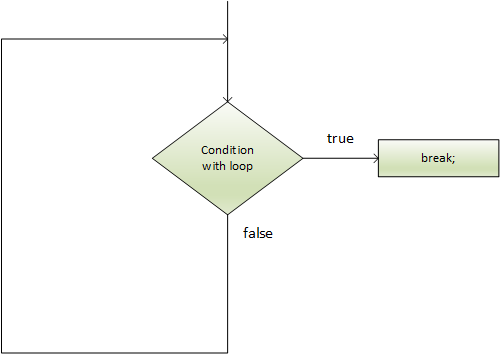# CS Break Statement

Break Statement is a declaration of the loop control that is used to end a loop. Once the break statement is found within a loop, the loop iterations stop and the controller immediately returns from the loop to the first declaration following the loop.

The C# break is used for breaking loop or switching statement. It interrupts the current program flow at the given stage. When the internal loop occurs, it only breaks the internal loop.

Syntax

``````
jump-statement;
break;

``````

Flowchart:Simple example of the C# break declaration used within the loop

``````
using System;
public class BreakExample
{
public static void Main(string[] args)
{
for (int i = 1; i <= 10; i++)
{
if (i == 6)
{
break;
}
Console.WriteLine(i);
}
}
}

``````

Output:

`1`
`2`
`3`
`4`
`5`

C# break declaration breaks the inner loop only, if we use break declaration within the inner loop.

``````
using System;
public class BreakExample
{
public static void Main(string[] args)
{
for (int i = 1; i<= 3; i++){
for (int j = 1; j<= 3; j++){
if (i == 2 && j == 2){
break;
}
Console.WriteLine(i + " " + j);
}
}
}
}

``````

Output:

`1 1`
`1 2`
`1 3`
`2 1`
`3 1`
`3 2`
`3 3`